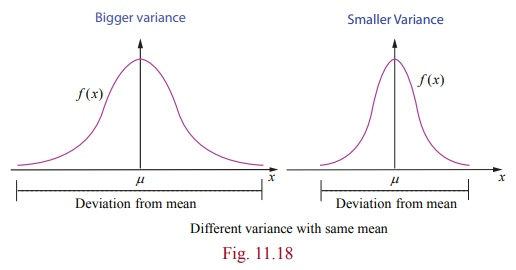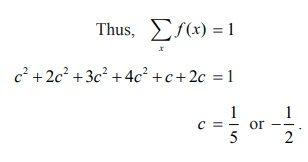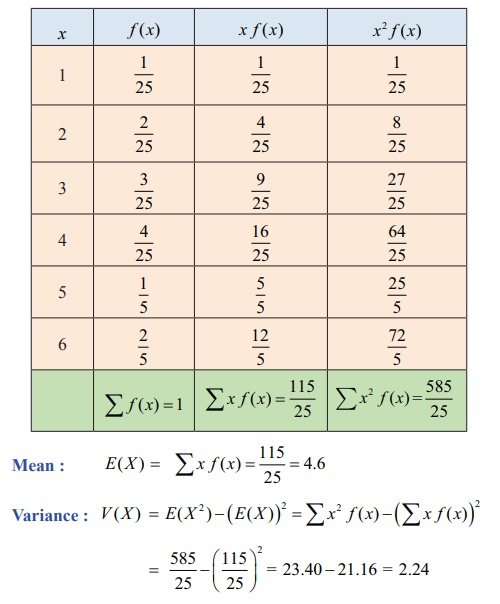Home | | Maths 12th Std | Properties of Mathematical expectation and variance

# Properties of Mathematical expectation and variance

Properties of Mathematical expectation and variance

Properties of Mathematical expectation and variance

(i) E(aX + b) = aE( X ) + b , where a and b are constants

Proof

Let X be a discrete random variableSimilarly, when X is a continuous random variable, we can prove it, by replacing summation by integration.(ii) Var ( X ) = E ( X2 ) −( E ( X ))2

### Proof

We know

E ( x) = μ

Var ( X ) = E (Xμ)2

= E (X2 – 2 + μ2)

= E (X2) – 2μE(X) + μ2

(Since μ is a constant)

= E (X2) – 2μμ + μ2 = E(X2)μ2

Var ( X ) = E (X2) – (E (X))2

An alternative formula to compute variance of a random variable X is

σ2 = Var ( X ) = E( X2) – (E( X ))2

(iii) Var(aX +b) = a2Var (X ) where a and b are constants

### Proof

Var ( aX + b) = E (( aX + b) E ( aX + b))2

= E ( aX + b aE( X ) b))2

= E (aX aE ( X )) 2

= E ( a ( X E( X )))2

= a2 E (XE( X ))2 .

Hence Var ( aX + b) = a2Var ( X )Variance gives information about the deviation of the values of the random variable about the mean μ. A smaller σ 2 implies that the random values are more clustered about the mean, similarly, a bigger σ 2 implies that the random values are more scattered from the mean.The above figure shows the pdfs of two continuous random variables whose curves are bell-shaped with same mean but different variances.

Example 11.16

Suppose that f ( x) given below represents a probability mass function,Find (i) the value of c (ii) Mean and variance.

Solution

(i) Since f ( x) is a probability mass function, f (x) 0 for all x , and ∑xf (x) = 1 .Since f (x) 0 for all x , the possible value of c is 1/5.

Hence, the probability mass function is(ii) To find mean and variance, let us use the following tableTherefore the mean and variance are 4.6 and 2.24 respectively.

### Example 11.17

Two balls are chosen randomly from an urn containing 8 white and 4 black balls. Suppose that we win Rs 20 for each black ball selected and we lose Rs10 for each white ball selected. Find the expected winning amount and variance.

### Solution

Let X denote the winning amount. The possible events of selection are (i) both balls are black, or (ii) one white and one black or (iii) both are white. Therefore x is a random variable that can be defined as

X (both are black balls) = 2(20) = 40

X (one black and one white ball) = 20 10 = 10

X (both are white balls) = (20) = − ₹ 20

Therefore X takes on the values 40,10 and 20

Total number of balls n = 12Example 11.18

Find the mean and variance of a random variable X , whose probability density function is### SolutionTags : Probability Distributions | Mathematics , 12th Maths : UNIT 11 : Probability Distributions
Study Material, Lecturing Notes, Assignment, Reference, Wiki description explanation, brief detail
12th Maths : UNIT 11 : Probability Distributions : Properties of Mathematical expectation and variance | Probability Distributions | Mathematics